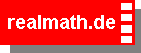To create a new problem,
click on the button  create problem .

 1 Adjust the first Fraction with NumeratorFraction1 and DenominatorFraction1, the second Fraction with NumeratorFraction2 and DenominatorFraction2. 2 Place the second Fraction over the first Fraction to visualize the renamed fractions and the answer. 3 Keep in mind that the violet part has to be counted twice when adding.

To validate your answer,
click on the button  validate .

Score:topic: Fractions
Adding fractions with unlike denominators (introduction)

 + = + =

© Andreas Meier, Weiden i.d.OPf.